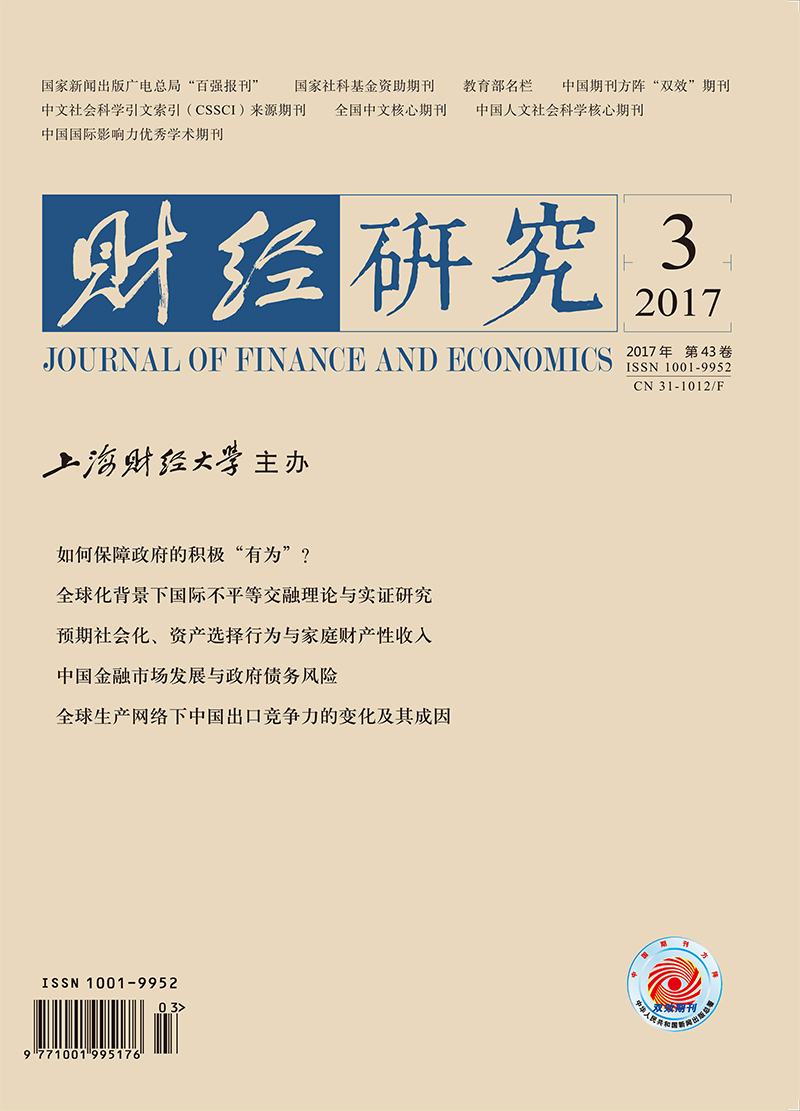﻿ 全球化背景下国际不平等交融理论与实证研究—来自中国贸易利益不平等的证据
 财经研究2017, Vol. 43Issue (3): 17-290

#### 文章信息财经研究2017年43卷第3期

Ma Yan, Wang Baozhu, Zhao Zhicheng, Li Jun.

Theoretical and Empirical Study on the Integration of International Inequality under the Background of Globalization: Evidence from Trade Benefit Inequalities in China

Journal of Finance and Economics, 2017, 43(3): 17-29.

### 文章历史《财经研究》
2017第43卷第3期

Theoretical and Empirical Study on the Integration of International Inequality under the Background of Globalization: Evidence from Trade Benefit Inequalities in China
Ma Yan, Wang Baozhu, Zhao Zhicheng, Li Jun
School of Economics, Shanghai University of Finance and Economics, Shanghai 200433, China
Abstract: Under the background of deepening economic globalization and constant changes in international division of labor, internal mechanism of international inequality issue has new changes and Marx's classical inequality theory is facing a novel challenge. Based on the new form of international division of labor, this paper puts the inequality in three fields of international trade, international investment and international finance into a unified "integration" framework, analyzes how international inequality transforms from inequality in a single field to integrated inequality in many fields, proposes the concept of international inequality integration, and further constructs a mathematical model of international trade interests inequality integration. Theoretical model shows that the inequality in an international economic field is integrated with inequality in other international forms, and is embodied in the increase or reduction in the transfer of international surplus value in an international economic field owing to the integration of other international economic activities in terms of quantity. Based on theoretical analysis above, this paper takes the international trade inequality as an example, and explores the problem of inequality integration in the three international economic fields. It comes to the results as follows:firstly, international investment inequality and relative differences in real effective exchange rates have a significant positive impact on international trade inequality; secondly, there does exist long-term bidirectional causality in inequality between international trade, international investment and international finance, namely there is an integration among these three. Therefore, the governments should examine different international economic fields on the same platform and reduce international inequality in a field accompanied by policy formulation in other two relative fields, to achieve a multiplier effect.
Key words: new form of international division of labor    international inequality    integration    international surplus value transformation

① 参见李长久：《对资本主义的几点认识》，《红旗文稿》，2012年第8期。原文为武者陵司：《世界进入空前的超额利润时代》，日本《经济学人》周刊，2007年5月8日。

20世纪50年代以前，国际分工主要表现为国别间的产品分工，而每一次工业革命都是对国际分工格局的一次重塑。如历史上第一次工业革命将资本主义社会带入了蒸汽时代，这同时也引致了第一次国际大分工—垂直分工形式。20世纪50年代至90年代，以跨国公司为主导，出现了产品内分工的新现象；分工理论也经历了绝对优势、比较优势、生产要素禀赋、新古典贸易、新新贸易等阶段。20世纪90年代之后，美国在制造业方面的优势逐步被日本和德国所代替。在新经济的比较优势逐渐弱化之后，相较于实体经济而言，美国在金融领域中的地位逐步凸显，并且将实体经济部门的资源逐步转向金融部门，即国际分工由产业内分工向产业间分工转变。

① 尽管2005年联合国贸易和发展会议(UNCTAD) 发表新闻公报宣布韩国进入发达国家之列，但由于韩国在人均GDPGDP增长率和人均国民总收入等方面与高收入发达国家均有显著的差距，因此，我们在这里将韩国作为发展中国家看待。就人均GDP而言，韩国2014年人均GDP为27 970.49美元，按照世界银行的分类，高收入国家为37 823.16美元(其中，北美为54 198.68美元，欧盟为39 567.18美元)，可见，韩国并未迈入高收入国家的行列，与美国、欧盟等发达地区的差距仍大。就GDP增长率而言，韩国2014年GDP增长率为3.31%，按照世界银行的分类，高收入国家增速为1.70%(其中，北美为2.4%，欧盟为1.29%)，韩国发展增速高于高收入国家，表明该经济体正处于向发达经济体收敛的进程中。就人均国民总收入GNI而言，韩国2014年GNI为34 620美元，按照世界银行的分类，高收入国家为40 762.47美元(其中，北美为54 622.89美元，欧盟为39 072.97美元)，可见，韩国并未迈入高收入国家的行列。

(一) 国际贸易与剩余价值转移

①这里并不要求欠发达国家生产的商品1全部用于出口，同样也不要求发达国家生产的商品2全部用于出口。

②其余商品只存在于国内市场。

 $\frac{1+r_{2}^{2}}{(1+{{{\bar{r}}}_{2}})q_{2}^{2}}(1+{{\bar{r}}_{1}})q_{1}^{2}-1\le r_{1}^{1}$ (1)

 $\frac{1+r_{2}^{2}}{1+r_{1}^{1}}\le \frac{1+{{{\bar{r}}}_{2}}}{1+\bar{r}}\times \frac{q_{2}^{2}}{q_{1}^{2}}$ (2)

 $\frac{1+r_{2}^{2}}{1+r_{1}^{1}}=\frac{1+{{{\bar{r}}}_{2}}}{1+\bar{r}}\times \frac{q_{2}^{2}}{q_{1}^{2}}$ (3)

 $r_{2}^{1}=\frac{1+r_{1}^{1}}{(1+{{{\bar{r}}}_{1}})q_{1}^{1}}(1+{{{\bar{r}}}_{2}})q_{2}^{1}-1~$ (4)

 ${{K}_{1}}(1+r_{1}^{1})+{{K}_{2}}(1+r_{2}^{2})={{K}_{1}}(1+{{{\bar{r}}}_{1}})+{{K}_{2}}(1+{{{\bar{r}}}_{2}})$ (5)

 ${{W}_{T}}={{K}_{1}}({{{\bar{r}}}_{1}}-r_{1}^{1})=\frac{(1-q_{1}^{2}/q_{2}^{2}){{K}_{1}}{{K}_{2}}(1+{{{\bar{r}}}_{1}})}{\lambda {{K}_{1}}q_{1}^{2}/q_{2}^{2}+{{K}_{2}}}$ (6)

(二) 国际投资对贸易不平等的影响

 $\frac{1+(r_{21}^{2})\prime }{1+(r_{1}^{1})\prime }=\frac{1+{{{\bar{r}}}_{2}}}{1+{{{\bar{r}}}_{1}}}\times \frac{q_{2}^{2}}{q_{1}^{2}}$ (7)

 $\frac{1+(r_{22}^{2})\prime }{1+{{{\bar{r}}}_{1}}}=\frac{1+(r_{21}^{2})\prime }{1+{{{\bar{r}}}_{2}}}$ (8)

 \begin{align} &{{K}_{1}}[1+(r_{1}^{1})\prime ]+({{K}_{2}}-\Delta K)[1+(r_{21}^{2})\prime ]+\Delta K[1+(r_{22}^{2})\prime ]= \\ &{{K}_{1}}(1+{{{\bar{r}}}_{1}})+({{K}_{2}}-\Delta K)(1+{{{\bar{r}}}_{2}})+\Delta K(1+{{{\bar{r}}}_{1}})~ \\ \end{align} (9)

 $W{{\prime }_{T}}={{K}_{1}}({{{\bar{r}}}_{1}}-(r_{1}^{1})\prime )=\frac{[{{K}_{2}}+(\lambda -1)\text{ }\Delta K](q_{2}^{2}-q_{1}^{2}){{K}_{1}}(1+{{{\bar{r}}}_{1}})}{\lambda {{K}_{1}}q_{1}^{2}+[{{K}_{2}}+(\lambda -1)\text{ }\Delta K]q_{2}^{2}}$ (10)

(三) 国际金融对贸易不平等的影响

 $(r_{1}^{1})\prime\prime \ge \theta \frac{1+(r_{2}^{2})\prime\prime }{(1+{{r}_{2}})q_{2}^{2}}(1+{{{\bar{r}}}_{1}})q_{1}^{2}-1$ (11)

 $\frac{1+(r_{2}^{2})\prime\prime }{1+(r_{1}^{1})\prime\prime }\le \frac{1+{{{\bar{r}}}_{2}}}{1+{{{\bar{r}}}_{1}}}\times \frac{q_{2}^{2}}{\theta q_{1}^{2}~}$ (12)

 $\frac{1+(r_{2}^{2})\prime\prime }{1+(r_{1}^{1})\prime\prime }=\frac{1+{{{\bar{r}}}_{2}}}{1+{{{\bar{r}}}_{1}}}\times \frac{q_{2}^{2}}{\theta q_{1}^{2}~}$ (13)

 ${{K}_{1}}(1+(r_{1}^{1})\prime\prime )+{{K}_{2}}(1+(r_{2}^{2})\prime\prime )={{K}_{1}}(1+{{{\bar{r}}}_{1}})+{{K}_{2}}(1+{{{\bar{r}}}_{2}})$ (14)

 $(r_{1}^{1})\prime\prime =\frac{{{K}_{1}}(1+{{{\bar{r}}}_{1}})+{{K}_{2}}(1+{{{\bar{r}}}_{2}})}{{{K}_{1}}(1+{{{\bar{r}}}_{1}})\theta q_{1}^{2}+{{K}_{2}}(1+{{{\bar{r}}}_{2}})q_{2}^{2}}(1+{{{\bar{r}}}_{1}})\theta q_{1}^{2}-1$ (15)
 $(r_{2}^{2})\prime\prime =\frac{{{K}_{1}}(1+{{{\bar{r}}}_{1}})+{{K}_{2}}(1+{{{\bar{r}}}_{2}})}{{{K}_{1}}(1+{{{\bar{r}}}_{1}})\theta q_{1}^{2}+{{K}_{2}}(1+{{{\bar{r}}}_{2}})q_{2}^{2}}(1+{{{\bar{r}}}_{2}})\theta q_{2}^{2}-1$ (16)

 $\Delta R={{K}_{1}}[(1+r_{1}^{1})-(1+(r_{1}^{1})\prime\prime )/\theta ]-{{K}_{2}}[(1+r_{2}^{2})-(1+(r_{2}^{2})\prime\prime )]$ (17)

 $\Delta R=2{{K}_{1}}(1+r_{1}^{1})-{{K}_{1}}(1+(r_{1}^{1})\prime\prime )(1+\theta )/\theta$ (18)

 \begin{align} &\Delta R=2{{K}_{1}}(1+{{r}_{1}})-[{{K}_{1}}(1+{{{\bar{r}}}_{1}})+{{K}_{2}}(1+{{{\bar{r}}}_{2}})] \\ &\left( 1+\frac{{{K}_{1}}(1+{{{\bar{r}}}_{1}})q_{1}^{2}-{{K}_{2}}(1+{{{\bar{r}}}_{2}})q_{2}^{2}}{{{K}_{1}}(1+{{{\bar{r}}}_{1}})\theta q_{1}^{2}+{{K}_{2}}(1+{{{\bar{r}}}_{2}})q_{2}^{2}~} \right) \\ \end{align} (19)

(1) 当发达国家各类产品的劳动生产率高于欠发达国家，且发达国家的出口商品生产者拥有垄断权利，而欠发达国家不具备这一权利时，国际分工和贸易会使欠发达国家的剩余价值被转移至发达国家，即产生了国际贸易不平等现象。

(2) 当发达国家将国内较落后的不存在技术溢出的生产环节转移至欠发达国家，且欠发达国家不对发达国家进行国际投资时，发达国家不仅可以通过国际投资直接从欠发达国家攫取剩余价值，而且可以通过国际贸易进一步转移欠发达国家企业生产的剩余价值。

(3) 以同一货币计价，当发达国家的出口总额小于欠发达国家的出口总额时，发达国家的货币升值使其贸易利益增加；相反，当发达国家的出口总额大于欠发达国家的出口总额时，发达国家的货币升值使其贸易利益减少。

(一) 计量模型构建与数据说明

 $\text{ln}TU{{E}_{it}}={{c}_{i}}+{{\beta }_{i1}}\text{ln}IU{{E}_{it}}+{{\beta }_{i2}}\text{ln}FU{{E}_{it}}+{{v}_{it}}~$ (20)

① 18个发达国家分别为澳大利亚、加拿大、丹麦、芬兰、法国、德国、希腊、冰岛、日本、荷兰、新西兰、挪威、葡萄牙、西班牙、瑞典、瑞士、英国和美国。

1.国际贸易不平等指标(TUE)。在现实经济中，国际贸易的不平等主要与发达国家和欠发达国家商品中所包含的劳动时间相关，这能够反映出发达国家从欠发达国家所获得的价值转移。等价交换意味着价值相同的商品所包含的劳动时间应该相同。发达国家与欠发达国家之间的劳动时间相对差异越大，国际贸易不平等就越大。因此，本文采用中国与各发达国家的单位价值出口商品所包含的劳动时间之比(TUE) 作为国际贸易不平等指标。由于无法精确度量出口商品所包含的劳动时间，我们假定总的活劳动的时间按照各商品净增加值占国内生产净值(NDP) 的比例进行分配，因此可以用单位净增加值商品所包含的劳动时间近似代替单位价值的出口商品所包含的劳动时间。鉴于数据的可获得性，本文用国民收入(NI) 代替国内生产净值进行计算。因此单位价值的出口商品所包含的劳动时间等于当年总劳动时间除以国民收入，前者等于就业总人数乘以人均每年劳动小时数。

GDP包含固定资产折旧，这部分是转移到产品中去的价值，并非活劳动所创造的价值。

③ 1991-2013年国民收入、各发达国家人均劳动小时和就业人数、中国就业人数等数据分别来源于世界银行数据库、OECD数据库、中经网统计数据库。2001-2013年中国人均劳动小时数据根据《中国劳动统计年鉴》中的“城镇就业人员调查周平均工作时间”计算而得。此外，1995年全国1%人口抽样调查资料统计显示，当年我国人均每周劳动时间为40.7小时。国际劳工组织2007年6月8日发布的研究报告也显示：中国1967-1984年人均每周劳动时间为48小时。因此取48小时与1995年人均每周劳动时间的平均值44.35小时作为1991-1994年人均每周劳动时间，取1995年和2001年人均每周劳动时间的平均值作为1996-2001年人均每周劳动时间。

2.国际投资不平等指标(IUE)。在现实经济中，国际投资的不平等主要与发达国家对欠发达国家的直接投资相关，这能够反映出发达国家在欠发达国家所获得收益的机会。当中国FDI流入存量相对于发达国家FDI流入存量越多时，表明其他国家尤其是发达国家在中国获取投资收益的机会相对更多；同时，中国FDI流出存量相对于发达国家FDI流出存量越少，表明中国在海外获取投资收益的机会相对更少。因此，本文采用中国与各发达国家FDI“流入-流出”存量比率之比(IUE) 作为衡量国际投资不平等的指标。

3.国际金融不平等指标(FUE)。在现实经济中，国际金融的影响是多方位的，反映国际金融影响的指标也是多样的，本文主要从汇率角度考虑国际金融作为制度因素的影响。因而国际金融的不平等主要与发达国家对欠发达国家的汇率相关，这能够反映出发达国家从欠发达国家所获取的贸易利益。因此，本文采用中国与各发达国家的实际有效汇率指数之比(FUE) 来度量国际金融不平等。

(二) 实证结果及其分析

1.单位根检验。为防止伪回归现象，首先需要对面板数据进行平稳性检验。为了保证平稳性检验结果的稳健性，本文选择多种面板数据单位根检验方法，并对这些检验方法进行综合考虑。结果(如表 1) 显示，lnTUE、lnIUE和lnFUE在四种检验方法中均不能在10%的显著性水平上拒绝“存在单位根”的原假设，因此这三个变量均为非平稳的。进行一阶差分处理后，这三个变量皆变为平稳序列，即同属于一阶单整变量。

 变量 LLC IPS ADF-fisher PP-fisher lnTUE -0.8650 -1.2002 39.711 13.435 lnIUE -0.7258 3.1279 12.108 10.125 lnFUE 2.2861 -0.7011 39.661 37.408 ΔlnTUE -7.6766*** -7.7664*** 122.69*** 113.57*** ΔlnIUE 10.674*** -9.1148*** 144.76*** 146.13*** ΔlnFUE -13.051*** -11.134*** 179.02*** 188.84*** 注：(1) ***、**和*分别表示通过1%、5%和10%的显著性水平检验，下同；(2) 变量lnTUE和lnIUE的检验形式为包含截距项和时间趋势项，其余变量的检验形式为只包含截距项；(3) 使用SIC准则选择滞后项。

 Pedroni检验 Kao检验 Panel ADF Group ADF ADF 统计量 -1.6569 -3.6995 -6.8716 P值 0.0488 0.0001 0.0000 注：(1) 三种检验方法的零假设为不存在协整关系，在零假设下统计量服从渐进正态分布；(2) 检验形式为只包含截距项，长期方差非参数估计方法采用Bartlett核函数。

3.面板协整方程的估计。为了描述国际投资不平等和国际金融不平等对国际贸易不平等的影响，本文采用Pedroni (2000) 的组间FMOLS方法和Pedroni (2000) 的组间DOLS方法对协整系数进行估计。结果(如表 3) 显示，两种估计方法对系数的估计结果较为接近，且高度显著。采用FMOLS方法进行估计时，lnIUE和lnFUE的系数分别为0.3852和2.5919，这表明当国际投资不平等和实际有效汇率相对差异各自扩大1%，将导致国际贸易不平等分别扩大0.38%和2.59%。采用DOLS方法进行估计时，lnIUE和lnFUE的系数分别为0.3329和2.7524，这表明国际投资不平等和实际有效汇率相对差异各自扩大1%，将导致国际贸易不平等分别扩大0.33%和2.75%。

 估计方法 lnIUE lnFUE Panel FMOLS(Pedroni，2000) 0.3852***(7.31) 2.5919***(10.68) Panel DOLS(Pedroni，2001) 0.3329*** (5.54) 2.7524***(7.61) 注：括号内为t统计量。

4.面板格兰杰因果关系检验。前面揭示了国际贸易不平等、国际投资不平等和国际金融不平等之间存在长期稳定的均衡关系，但尚不清楚各变量之间的格兰杰因果关系。由于lnTUE、lnIUE和lnFUE之间存在协整关系，对三者的因果关系检验不能运用差分VAR模型，而应采用面板向量误差修正模型(PVECM)：

 $\begin{array}{l} \left[ \begin{array}{l} \Delta {\rm{ln}}TU{E_{it}}\\ \Delta {\rm{ln}}IU{E_{it}}\\ \Delta {\rm{ln}}FU{E_{it}} \end{array} \right] = \left[ \begin{array}{l} {\varphi _{1i}}\\ {\varphi _{2i}}\\ {\varphi _{3i}} \end{array} \right] + \sum\limits_{j = 1}^p {\left[ {\begin{array}{*{20}{c}} {{\varphi _{11j}}}&{{\varphi _{12j}}}&{{\varphi _{13j}}}\\ {{\varphi _{21j}}}&{{\varphi _{22j}}}&{{\varphi _{23j}}}\\ {{\varphi _{31j}}}&{{\varphi _{32j}}}&{{\varphi _{33j}}} \end{array}} \right]} \\ \left[ \begin{array}{l} \Delta {\rm{ln}}TU{E_{it - j}}\\ \Delta {\rm{ln}}IU{E_{it - j}}\\ \Delta {\rm{ln}}FU{E_{it - j}} \end{array} \right] + \left[ \begin{array}{l} {\lambda _1}\\ {\lambda _2}\\ {\lambda _3} \end{array} \right]{{\hat v}_{it - 1}} + \left[ \begin{array}{l} {\xi _{1it}}\\ {\xi _{2it}}\\ {\xi _{3it}} \end{array} \right] \end{array}$ (21)

 被解释变量 短期因果关系 长期因果关系 ΔlnTUE ΔlnIUE ΔlnFUE $\hat{v}$-1 $\hat{v}$-1/ΔlnTUE $\hat{v}$-1/ΔlnIUE $\hat{v}$-1/ΔlnFUE ΔlnTUE 6.31**[0.0427] 8.80**[0.0123] -0.2232***(-4.11) 39.34***[0.0000] 29.32***[0.0000] ΔlnIUE 12.85***[0.0016] 30.71***[0.0000] 0.1291**(2.57) 24.35***[0.0000] 46.21***[0.0000] ΔlnFUE 18.19***[0.0001] 10.94***[0.0042] 0.3021***(3.32) 19.10***[0.0003] 50.94***[0.0000] 注：(1) 为了消除序列相关，模型的最优滞后阶数为2阶；(2) 模型通过了序列相关检验和Sargan检验；(3) $\hat{v}$-1/X表示对误差修正项$\hat{v}$-1和滞后解释变量X进行联合检验；(4) 系数值为卡方统计量，中括号内为p值，圆括号内的数值为z统计量。

*感谢上海财经大学优秀博士学位论文培育(第七批)基金资助项目的支持。

  陈同仇, 薛荣久. 国际贸易[M]. 北京: 对外经济贸易大学出版社, 1997.  程恩富, 夏晖. 美元霸权:美国掠夺他国财富的重要手段[J]. 马克思主义研究, 2007(12): 28–34.  雷达, 赵勇. 中美经济失衡的性质及调整:基于金融发展的视角[J]. 世界经济, 2009(1): 62–71.  李真. 经济全球化条件下我国贸易利益影响因素分析—基于技术进步和国际制度视角的考察[J]. 马克思主义研究, 2009(12): 76–85.  李真, 马艳. 贸易不平等交换的多因素诱因模型与实证研究—来自于多国面板的证据[J]. 财贸经济, 2011(4): 96–103.  廖泽芳, 雷达. 全球经济失衡的利益考察—基于估值的视角[J]. 世界经济研究, 2012(9): 3–10.  聂志红. 经济全球化下的不平等交换逻辑[J]. 社会科学研究, 2008(5): 24–28.  齐昊. 马克思主义的不平等交换理论与中国对外贸易的现实[J]. 政治经济学评论, 2008(1): 64–85.  萨米尔·阿明. 不平等的发展—论外围资本主义的社会形态[M]. 北京: 商务印书馆, 1990.  王厚双, 李艳秀. 全球经济失衡与全球经济再平衡研究新进展[J]. 经济学家, 2015(3): 84–92.  王岚, 盛斌. 全球价值链分工背景下的中美增加值贸易与双边贸易利益[J]. 财经研究, 2014(9): 97–108.  王元璋, 阮红新. 论国际市场的不等价交换—谈对陈同仇、薛荣久两先生"国际不等价交换观"的一点看法[J]. 理论月刊, 2000(3): 31–32.  徐建炜, 姚洋. 国际分工新形态、金融市场与全球失衡[J]. 世界经济, 2010(3): 3–30.  伊曼纽尔. 不平等交换[M]. 北京: 中国对外经济贸易出版社, 1988.  约翰·罗默.在自由中丧失[M].段忠桥、刘磊译, 经济科学出版社, 2003.  张茉楠. 国际分工视角下的全球经济失衡与利益分配格局调整[J]. 金融与经济, 2012(5): 10–18.  朱奎. 等价交换中的不平等问题研究[J]. 学习与探索, 2006(5): 209–212.  Findlay R. The terms of trade and equilibrium growth in the world economy[J]. The American Economic Review, 1980, 70(3): 291–299.  Krugman P R. A model of innovation, technology transfer, and the world distribution of income[J]. The Journal of Political Economy, 1979, 87(2): 253–266. DOI:10.1086/260755  Krugman P R. Trade accumulations and uneven development[J]. Journal of Development Economics, 1981, 8(2): 149–161. DOI:10.1016/0304-3878(81)90026-2  Pan-Long T. Foreign direct investment and income inequality:Further evidence[J]. World Development, 1995, 23(3): 469–483. DOI:10.1016/0305-750X(95)00136-Z  Pedroni P. Fully modified OLS for heterogeneous cointegrated panels[J]. Advances in Econometrics, 2001, 15: 93–130.  Pedroni P. Purchasing power parity tests in cointegrated panels[J]. Review of Economics & Statistics, 2001, 83(4): 727–731.  Phillips P C B, Hansen B E. Statistical inference in instrumental variables regression with I (1) processes[J]. The Review of Economic Studies, 1990, 57(1): 99–125. DOI:10.2307/2297545